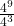## Simplify this expression.Question

Simplify this expression.in progress 0
5 days 2021-07-20T10:38:58+00:00 2 Answers 2 views 0

4⁹ / 4³ = 4⁶ = 4096

Step-by-step explanation:

You can solve this by realising that exponents (the 9 and the 3 in this case), simply tell you how many times to multiply the numbers together.

What you’re see there then can also be expressed as:Because the numerator and denominator are all multiples of the same number repeatedly, you can simply eliminate the bottom three fours from the top and bottom, giving you:A simpler way to look at it is to understand that when the same number is being raised to different bases, and then multiplied or divided, you can simply add or subtract the exponents.  For example, speaking very generically:andIn this case then, we can simply say:So when simplified, the expression is equal to 4⁶, or 4096.

2. 9x9x9x9divide by 4x4x4

3.